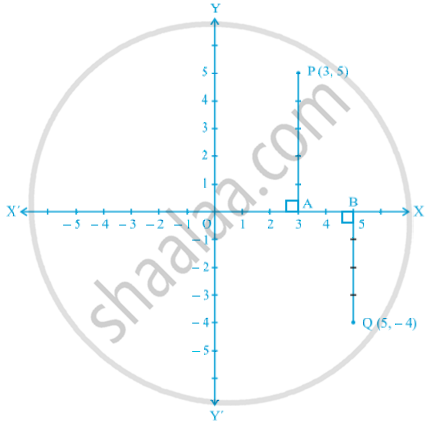# Plotting a Point in the Plane If Its Coordinates Are Given.

#### notes

Let the coordinates of a point be (3, 5). We want to plot this point in the coordinate plane. We draw the coordinate axes, and choose our units such that one centimetre represents one unit on both the axes. The coordinates of the point (3, 5) tell us that the distance of this point from the y - axis along the positive x - axis is 3 units and the distance of the point from the x - axis along the positive y - axis is 5 units. Starting from the origin O, we count 3 units on the positive x - axis and mark the corresponding point as A. Now, starting from A, we move in the positive direction of the y - axis and count 5 units and mark the corresponding point as P as the following fig.The distance of P from the y - axis is 3 units and from the x - axis is 5 units. Hence, P is the position of the point. Note that P lies in the 1st quadrant, since both the coordinates of P are positive. Similarly, you can plot the point Q (5, – 4) in the coordinate plane. The distance of Q from the x - axis is 4 units along the negative y - axis, so that its y - coordinate is – 4 as above fig. The point Q lies in the 4th quadrant.

If you would like to contribute notes or other learning material, please submit them using the button below.

### Shaalaa.com

Plotting coordinates of a point in the plane Graph [00:03:19]
S
0%